# NEET Important Chapter - Alternating Current

Get plus subscription and access unlimited live and recorded courses## Alternating Current: An Important Concept for NEET

The chapter of Alternating Current starts with the basic revision of all the concepts we have studied in our lower grades and helps to build a connection between the advanced parts of Alternating Current. In the following chapter, we have discussed alternating and direct current, average and rms value of alternating current, resistance offered by various elements (inductor, resistor and capacitor) to a.c , impedances and phases of ac circuits containing different elements, etc.

While deriving the formulae and equations for resistance offered by various elements (inductor, resistor and capacitor) to a.c., L.C Oscillations, we will also study about transformers and the power losses in a transformer with examples in the chapter Alternating Current.

In this chapter, students will also get to learn about the Power in AC Circuit and Resonance, where we will study about the sharpness of resonance  along with other major topics.

Now, let us move on to the important concepts and formulae related to NEET  exams along with a few solved examples.

### Important Topics of Alternating Current

• Alternating Current

• Impedance

• Average and RMS value of AC

• Reactance

• Resonance

• Power Factor

• L. C. Oscillations

• Transformer

### Important Concepts of Alternating Current

 Sl.No Name of the Concept Key Points of the Concept 1. Alternating Current and Direct Current If a current periodically changes its direction and  magnitude then it is termed as alternating current. The change can be seen as it first increases from zero to a maximum value, then decreases to zero and reverses in direction, increases to a maximum in this direction and then decreases to zero.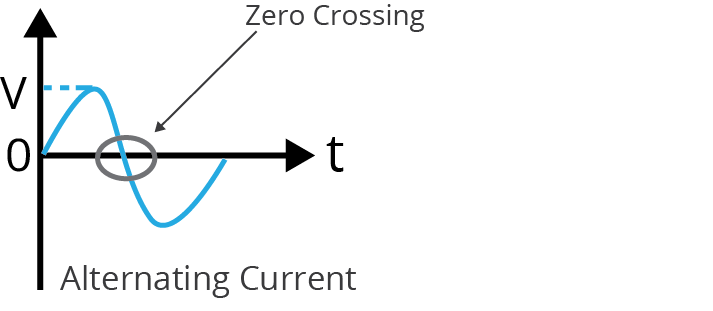On the other hand, direct current is that which can change the magnitude but never changes its direction.(image will be uploaded soon) 2. Average and RMS value of current The average value for an AC cycle is zero because of equal positive and negative cycles.The rms value of AC is defined as the direct current producing the same heating effect in a given resistor in a given time as is produced by the given A.C. through the same resistor for the same time period.$I_{rms} = \dfrac{I_{0}}{\sqrt{2}}$ 3. AC Circuit Containing Resistance Only For a pure resistive circuit where only the resistor is connected in the circuit with some alternating voltage source $E = E_{m}\sin(wt)$ and current $i= i_{m}\sin(wt)$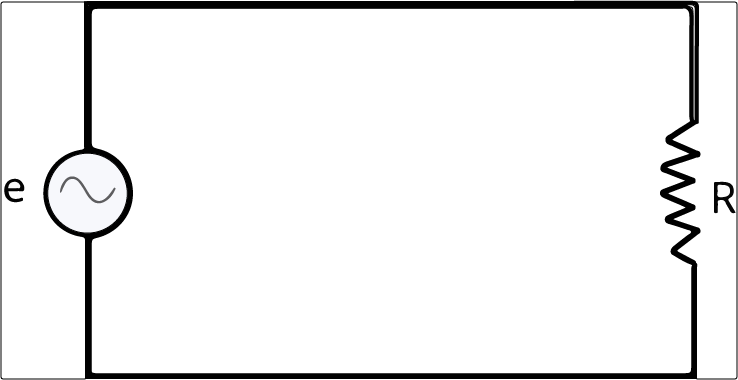Current and voltage here are in phase.(image will be uploaded soon)Impedance of the circuit here is the resistance only. 4. AC Circuit Containing Inductor Only For a pure inductive circuit where only the inductor L is connected in the circuit with some alternating voltage source $v = v_{m}\sin(wt)$,the current flowing through the inductor circuit is $i = i_{m}\sin(wt - \dfrac{\pi}{2})$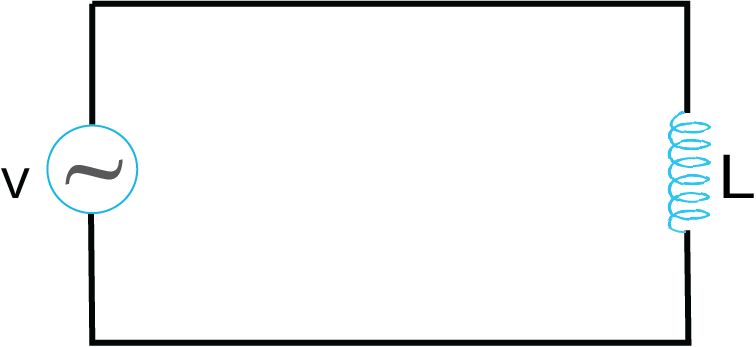Current in this circuit lags behind the applied voltage source by phase difference of $\dfrac{\pi}{2}$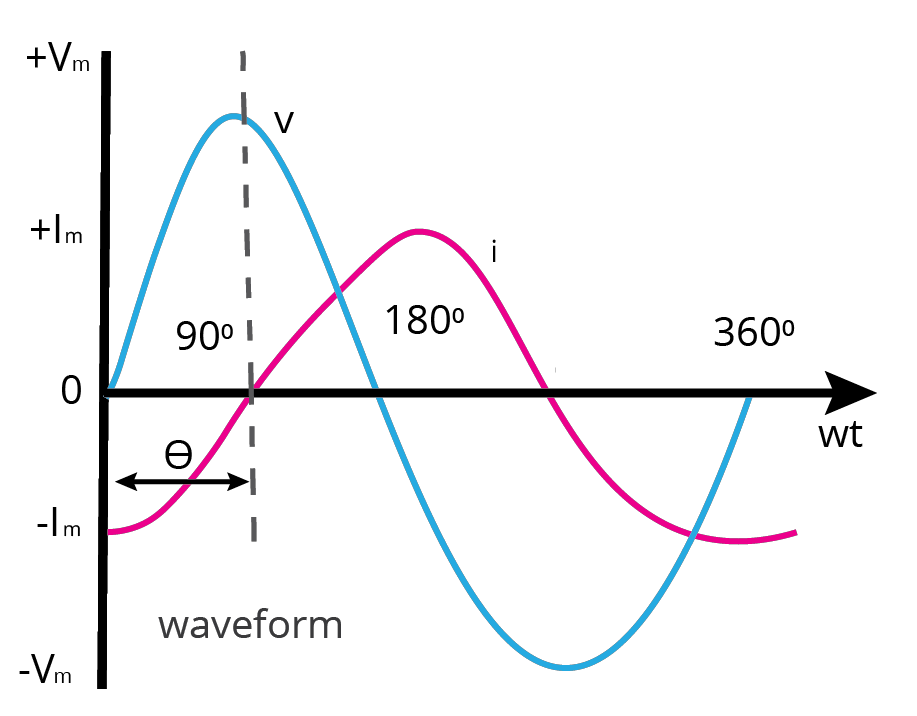Impedance of the circuit is called inductive reactance = wL 5. AC Circuit Containing Capacitor Only For a pure capacitive circuit where only the capacitor C is connected in the circuit with some alternating voltage source $v = v_{m}\sin(wt)$,the current flowing through the capacitor circuit is $i = i_{m}\sin(wt + \dfrac{\pi}{2})$Current in this circuit leads the applied voltage source by phase difference of $\dfrac{\pi}{2}$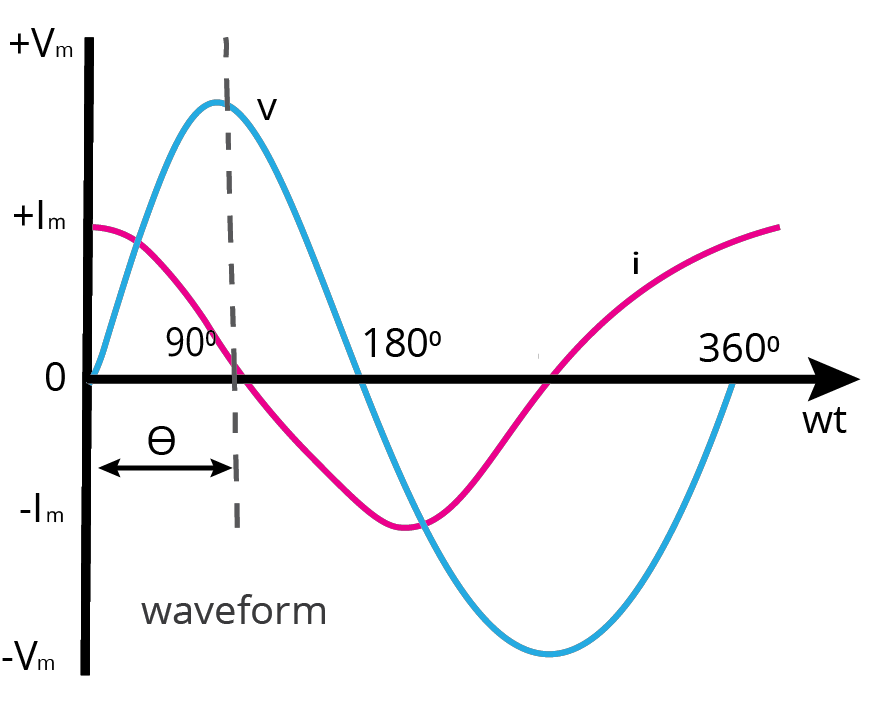Impedance of the circuit is called capacitive reactance = 1/wC 6. AC Circuit Containing Resistor, Inductor and Capacitor in series For a series circuit where resistor, inductor and capacitor are connected in series in the circuit with some alternating voltage source $v = v_{m}\sin(wt)$the current flowing through the series circuit is $i = i_{m}\sin(wt +\phi)$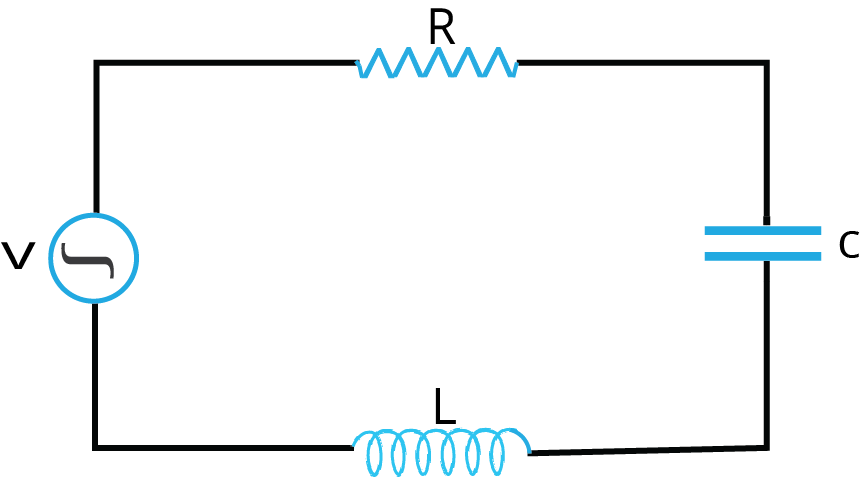Here the impedance of the circuit is $Z = \sqrt{R^{2} + (X_{L}- X_{C})^{2}}$ And Phase = $\tan\phi = \dfrac{X_{L} - X_{C}}{R}$ 7. Resonance Resonance is one of the main characteristics of an LCR circuit which means that if a particular frequency matches with the natural frequency of the system then that condition is called resonance.$w_{o}$ =$\dfrac{1}{\sqrt{LC}}$ this $w_{o}$ is called resonant frequency. 8. Power in AC Circuit In AC circuit, power for one cycle is defined as the rate of doing work hence if $v = v_{m}\sin(wt)$Current $i$=$i_{m}\sin(wt +\phi)$Then power = $P = v\times i$ 9. Transformer A device which is used for converting either high voltage to low voltage or vice versa is called a transformer.A transformer is classified into two categories:Step-up TransformerStep-down Transformer

### List of Important Formulae

 Sl. No Name of the Concept Formula 1. Rms value of current $I_{rms} = \dfrac{I_{0}}{\sqrt{2}}$ 2. Inductive Reactance $X_{L} = {\omega L}$ 3. Capacitive Reactance $X_{C} = \dfrac{1}{\omega C}$ 4. Impedance of LCR Circuit $Z = \sqrt{R^{2} + (X_{L}- X_{C})^{2}}$ 5. Resonance $w_{o} =\dfrac{1}{\sqrt{LC}}$ this $w_{o}$ is called Resonant frequency.At resonance, Z is minimum and current is maximum i.e.$I_{max} =\dfrac{V}{Z_{min}}$ 6. Power in AC Circuit Power = $P = v\times i$ 7. Efficiency of Transformer $\dfrac{E_{S}\times I_{S}}{E_{P}\times I_{P}}$Here $E_{S}$, $I_{S}$ are the output voltage and current of secondary coil and $E_{P}$, $I_{P}$ are the input voltage and current of the primary coil.

### Solved Examples of Alternating Current

1. The current in an inductive circuit is given by 0.3 sin (200t – 40°) A. Write the equation for the voltage across it if the inductance is 40 mH.

Sol:

Given, i = 0.3 sin (200t – 40°) A, L = 40mH  =$40 \times 10^{-3} H$

$X_{L}$ = ${\omega L}$ = $200\times 400 \times 10^{-3}$ = 8Ω

$V_{m}$ = $I_{m}\times X_{L}$ = $0.3 \times 8$ =2.4V

In an inductive circuit, the voltage leads the current by 90^{\circ}.

Therefore,

V = $V_{m}sin(\omega\times t + 90^{\circ})$

V = $2.4 sin(200t - 40 ^{\circ} + 90^{\circ})$

V = $2.4 sin(200t + 50^{\circ})$.

Key point: The ques here focuses mainly on the phase difference between current and voltage in an inductive circuit.

2. The output of a step-down transformer is measured to be 24 V when connected to a 12 W light bulb. The value of the peak current is

1. $\dfrac{1}{\sqrt{2}}$

2. $\sqrt{2}A$

3. $2A$

4. $2 \sqrt{2}A$

Sol:

Given:

Output of Secondary Voltage = $V_{s}$ = 24V

Power associated with Secondary $P_{s}$ = 12W

$I_{s}$ = $\dfrac{P_{s}}{V_{s}}$ = $\dfrac{12}{24}$ = 0.5A

Amplitude of the current in the secondary winding:

$I_{\circ}$ = $I_{\circ}$ = $I_{s}\sqrt{2}$

= 0.5(1.414) = 0.707 = $\dfrac{1}{\sqrt{2}}$A.

Hence, Option (a) is Correct.

Key Point: Here, in the case of a step-down transformer, the output voltage decreases and the output current increases.

### Previous Year Questions of Alternating Current

1. An inductor 20 mH, a capacitor 50μF and a resistor 40Ω are connected in series across a source of emf  V=10 sin 340t. The power loss in A.C. circuit is (NEET - 2016)

1. 0.51 W

2. 0.67 W

3. 0.76 W

4. 0.89 W

Sol:

Given:

V=10 sin 340t = $V_{rms} = \dfrac{10}{\sqrt{2}}$

L = 20mH = $20 \times 10^{-3} H$

C = 50μF = $50 \times 10^{-6} F$

R = 40Ω and V=10 sin 340t

$X_{C}$ =$\dfrac{1}{\omega C}$ = $X_{C}$ = $\dfrac{1}{340\times 50 \times 10^{-6}}$ = 58.8Ω

$X_{L} = {\omega L}$ =  ${340\times 20 \times 10^{-3} }$ = 6.8Ω

$Z = \sqrt{R^{2} + (X_{L}- X_{C})^{2}}$ = $Z = \sqrt{40^{2} + (58.8- 6.8)^{2}}$ = $\sqrt{4304}$Ω

Hence P = $i_{rms}^{2}\times R$

Or P = $(\dfrac{V_{rms}}{Z})^{2}\times R$

=$(\dfrac{50}{4304}) \times 40$  = 0.47W

Correct solution is 0.47W and the closest option is (a).

Trick: Here the formulae of capacitive, inductive reactance and impedance play a major role in ans along with the power.

3. A series LCR circuit is connected to an AC voltage source. When L is removed from the circuit, the phase difference between current and voltage is $\dfrac{\pi} {3}$. If instead C is removed from the circuit, the phase difference is again $\dfrac{\pi} {3}$ between current and voltage. The power factor of the circuit is  (NEET 2020)

1. 0.51W

2. 0.67W

3. 0.76W

4. 0.89 W

Sol:

Given:

When L is removed,

Phase = $\tan\phi$= $\dfrac{ X_{C}}{R}$ = $\tan\dfrac{\pi}{3}$ = $\dfrac{\ X_{C}}{R}$

When C is removed,

Phase=$\tan\phi$ = $\dfrac{X_{L}}{R}$ = $\tan\dfrac{\pi}{3}$ = $\dfrac{\ X_{L}}{R}$

From both above-mentioned equations, we have $X_{C} = X_{L}$

Hence, the circuit is in resonance and Z = R

So, power factor = $\cos\phi=\dfrac{R}{Z}$

The correct answer is 1 and option is b.

Trick: Formula of phase is given as = $\tan\phi = \dfrac{X_{L} - X_{C}}{R}$

### Practice Questions

1. A 100 mH inductor, a 25 μF capacitor and a 15 Ω resistor are connected in series to a 120 V, 50 Hz a.c. source. Calculate

(a) impedance of the circuit at resonance.

(b) current at resonance.

(c) Resonant frequency.

(Ans (a) 15 Ω (b) 8 A (c) 100.7 Hz)

2. The inductance of a choke-coil is 0.2 Henry and its resistance is 0.50 Ω . If a current of 2.0 ampere (rms value) and frequency 50Hz is passed through it, what will be the potential difference across its ends ?

(Ans: 125.6 V)

### Conclusion

In conclusion we can say that the chapter Alternating Current has covered all the important formulae and concepts like Power factor, Impedance, Reactance offered by different electrical circuits, etc. which will help in boosting your confidence to score high marks in the NEET 2022 exam. Students can have hands-on experience of the exam by practising the numericals.

See More## NEET Important Dates

View All Dates
NEET 2022 exam date and revised schedule have been announced by the NTA. NEET 2022 will now be conducted on 17-July-2022, and the exam registration closes on 20-May-2022. You can check the complete schedule on our site. Furthermore, you can check NEET 2022 dates for application, admit card, exam, answer key, result, counselling, etc along with other relevant information.
See More
View All Dates## NEET Information

Application Form
Eligibility Criteria
Reservation Policy
NTA has announced the NEET 2022 application form release date on the official website https://neet.nta.nic.in/. NEET 2022 Application Form is available on the official website for online registration. Besides NEET 2022 application form release date, learn about the application process, steps to fill the form, how to submit, exam date sheet etc online. Check our website for more details.## NEET 2022 Study Material

View NEET Syllabus in Detail
View NEET Syllabus in Detail## NEET 2022 Study Material

View all study material for NEET
All
Physics
Chemistry
Biology
See All## NEET Question Papers## NEET 2022 Book Solutions and PDF Download

View all NEET Important Books
Biology
NCERT Book for Class 12 Biology
Physics
NCERT Book for Class 12 Physics
Chemistry
NCERT Book for Class 12 Chemistry
Physics
H. C. Verma Solutions
See All## NEET Mock Tests

View all mock tests
"NEET 2022 free online mock test series for exam preparation are available on the Vedantu website for free download. Practising these mock test papers of Physics, Chemistry and Biology prepared by expert teachers at Vedantu will help you to boost your confidence to face the NEET 2022 examination without any worries. The NEET test series for Physics, Chemistry and Biology that is based on the latest syllabus of NEET and also the Previous Year Question Papers."
See More## NEET 2022 Cut Off

NEET Cut Off
NTA is responsible for the release of the NEET 2022 cut off score. The qualifying percentile score might remain the same for different categories. According to the latest trends, the expected cut off mark for NEET 2022 is 50% for general category candidates, 45% for physically challenged candidates, and 40% for candidates from reserved categories. For the general category, NEET qualifying marks for 2021 ranged from 720-138 general category, while for OBC/SC/ST categories, they ranged from 137-108 for OBC, 137-108 for SC and 137-108 for ST category.
See More## NEET 2022 Results

The NEET 2022 result is published by NTA on https://neet.nta.nic.in/ in the form of a scorecard on 7-Sep-2022. The scorecard will include the roll number, application number, candidate's personal details, and the percentile, marks, and rank of the candidate. Only those candidates who achieve the NEET cut-off will be considered qualified for the exam.
See More
Rank List
Counselling
Cutoff
NEET 2022 state rank lists will be released by the state counselling committees for admissions to the 85% state quota and to all seats in private medical and dental colleges. NEET 2022 state rank lists are based on the marks obtained in entrance exams. Candidates can check the NEET 2022 state rank list on the official website or on our site.## NEET Top Colleges

View all NEET 2022 Top Colleges
Want to know which Engineering colleges in India accept the NEET 2022 scores for admission to Engineering? Find the list of Engineering colleges accepting NEET scores in India, compiled by Vedantu. There are 1622 Colleges that are accepting NEET. Also find more details on Fees, Ranking, Admission, and Placement.
See More## FAQs on NEET Important Chapter - Alternating Current

FAQ

1. What is the weightage of the Alternating Current in NEET?

Nearly 1-2 questions appear in the exam from this chapter, covering about 10 marks which makes about 2% of the total marks.

2. What are the key points that need to be practised for solving questions from Alternating Current ?

Students should practice enough numericals and learn formulae for solving the questions from the Alternating Current .

3. Are previous year questions enough for NEET ?

Aspirants should know that revision is the key factor to any preparation for NEET. Candidates can easily evaluate their NEET preparation and work on the portion they need to and try to overcome lackings. As famously said, practice is the key to success so more practice = 600+ marks in the exam.## Notice board

News
BlogTrending pages